# Pyspark DataFrame

Spark is indeed a data analytics platform that's mostly used for analyzing enormous amounts of data. It enables us to distribute data and computational tasks over multiple clusters, resulting in a significant performance boost. Currently, Data Scientists tend to favor Spark over certain other information processing techniques because of its numerous advantages. The cost of data gathering, storage, & transmission is reduced when Spark is used. While working on a real-world problem, we're going to have a lot of data to deal with. As a result, distributed engines such as Hadoop, Spark, and others are quickly becoming the most important tools in the data science ecosystem. In this article, we will discuss Pyspark, Pyspark DataFrame, its functions, different ways to create Pyspark Dataframe. Let's go through them in detail.

Pyspark Dataframe - Table of Content

### What is Pyspark:

PySpark is a Python-based data analytics tool designed by the Apache Spark Community to be used with Spark. In Python, it enables us to interact with RDDs (Resilient Distributed Datasets) and Data Frames. When it comes to dealing with large amounts of data, PySpark gives us rapid flexibility, real-time processing, in-memory calculation, and a variety of additional characteristics. This is a Python library for using Spark that blends the Python language's ease with Spark's effectiveness.

## What is Pyspark Data frame:

A Data Frame is a networked collection of information that is organized in rows by named columns. In basic terms, this is similar to a table in a relational database or a column header in an Excel spreadsheet. Data Frames are primarily intended for handling vast amounts of organized or semi-structured information.

For this data analysis, we're utilizing Google Collab as our IDE. To begin, we must first install PySpark on Google Collab. Then we'll install the pyspark.sql package and build a Spark Session, which will be the Spark SQL API's point of entry.

`#installing pyspark!pip install pyspark#importing pysparkimport pyspark#importing spark sessionfrom pyspark.sql import Spark Session#creating a spark session object and providing App Name spark=SparkSession.builder.appName("pysparkdf").getOrCreate()`

The SparkSession object would communicate with Spark SQL's procedures and functions. Let's generate a Spark DataFrame from a CSV file now. We'll use a basic dataset from Kaggle called Nutrition Data on 80 Cereal Products.

Start learning PySpark training from hkrtrainings to make a bright career in the world of PySpark!

`#creating a dataframe using spark object by reading csv filedf = spark.read.option("header", "true").csv("/content/cereal.csv")#show df created top 10 rowsdf.show(10)`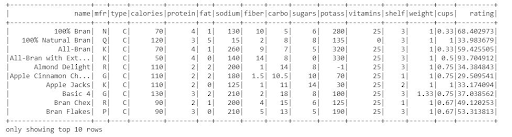That would be the Dataframe we'll use to analyse the data. Let's print the DataFrame's structure to learn a little about the dataset.

`df.printSchema()`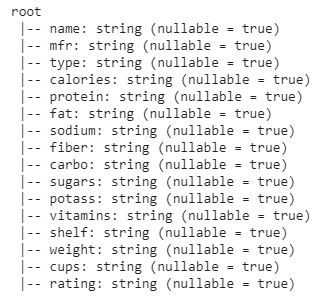DataFrame includes 16 columns or features. Each column includes string-type values.

### Functions of Pyspark Dataframe:

The functions of Pyspark Data frame are as follows:

select(): We may use the select function to show a collection of selected columns out of an entire data frame by only passing the column names. Let's use select() to print any of the three columns from the data frame.

`df.select('name', 'mfr', 'rating').show(10)`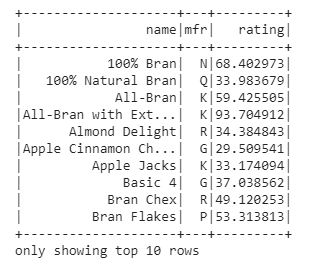We obtained a subset of the data frame with three columns: mfr, name, and rating in the output.

#### withColumn():

The with column function is utilized to alter a column or to generate a new column from one that already exists. We could also modify the datatype of every existing column using this transformation technique.

We can see that all of the columns in the DataFrame structure are of the string type. Now we can alter the calorie column's type of data to an integer.

`df.withColumn("Calories",df['calories'].cast("Integer")).printSchema()`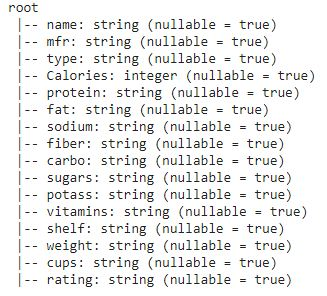The Datatype of the calories column has been converted to an integer type in the schema.

#### groupBy():

On DataFrame, the groupBy() function collects data into clusters and lets us apply aggregate operations on the dataset. This is a typical data analysis technique that is analogous to the SQL groupBy clause.

Let's count how many of each cereal there is in the dataset.

`df.groupBy("name", "calories").count().show()`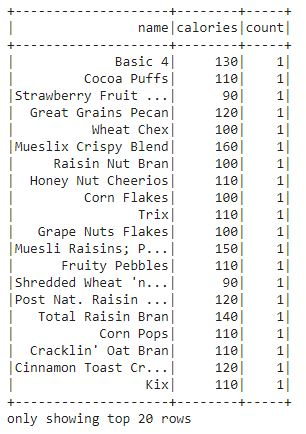#### orderBy():

The orderBy function is utilized to arrange the complete data frame depending on one of the data frame's columns. It arranges the data frame's rows according to on column values. It classifies in ascending order by default.

Let's create a data frame depending on the dataset's protein column.

`df.orderBy("protein").show()`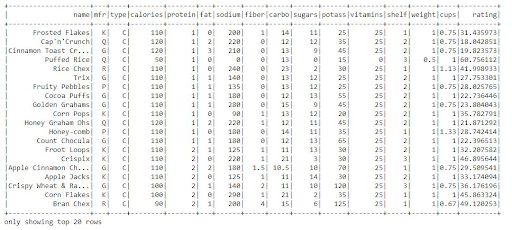The whole data frame is categorized by the protein column.

#### split():

The split() function divides a data frame's string column into numerous columns. Using the assistance of Column() and select(), this method is designed for the data frame.

The data frame's name column indicates values within two string words. From the gap between two strings, let's separate the name columns into two columns.

fropm pyspark.sql.functions import split

`df1 = df.withColumn('Name1', split(df['name'], " ").getItem(0))        .withColumn('Name2', split(df['name'], " ").getItem(1))df1.select("name", "Name1", "Name2").show()`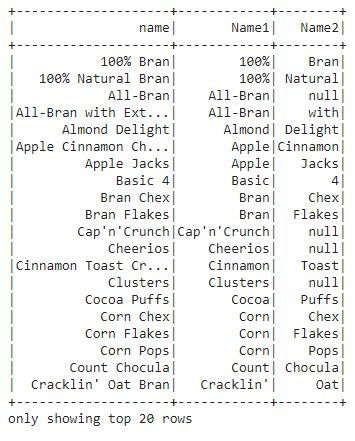We could find that the name column has been split into columns in this output.

#### lit():

lit() adds a new column to a data frame which includes literals or a constant value. Let's include a column called "intake quantity" that comprises a constant value for every cereal as well as the name of the cereal.

from pyspark.sql.functions import lit

`df2 = df.select(col("name"),lit("75 gm").alias("intake quantity"))df2.show()`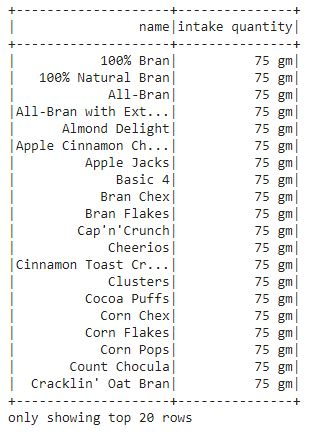We can observe that a new column "intake quantity" is produced in the output, which provides the intake an amount of every cereal.

We have the perfect professional PySpark Tutorial for you. Enroll now!

#### when():

The when() method is utilized to display data depending on the circumstance. It assesses the criteria and afterward gives the values based on the results. It's a SQL function that PySpark can use to check many conditions in a row and produce a result. The function operates in the same way that even if and switch statements do.

Let's have a look at some vitamin-rich cereals.

from pyspark.sql.functions import when

`df.select("name", when(df.vitamins >= "25", "rich in vitamins")).show()`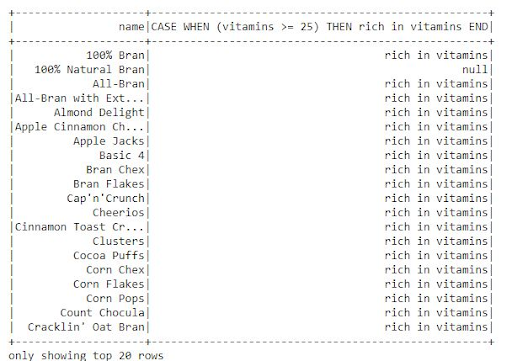#### Filter():

The filter function is utilized to classify data in rows according to the values of specific columns. We can, for example, filter cereals with a calorie count of 100.

from pyspark.sql.functions import filter

`df.filter(df.calories == "100").show()`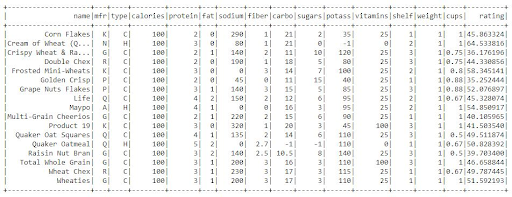We could see that the information has been filtered to only include cereals with 100 calories in this result.

#### isNull()/isNotNull():

`isNull()/isNotNull():#isNotNull()from pyspark.sql.functions import *#filter data by null valuesdf.filter(df.name.isNotNull()).show()`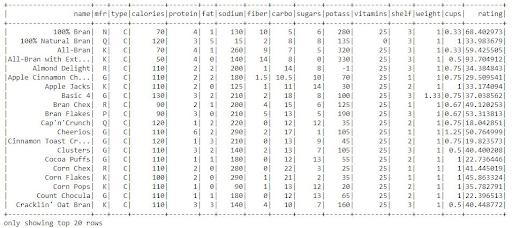These two functions are used to determine if the DataFrame has any null values. It is the most important data processing function. It is the most commonly used data cleansing tool.

Let's see whether there are any null values in the dataset.

In this dataset, there are no null values. As a result, the complete data frame is accessible.

`isNull():df.filter(df.name.isNull()).show()`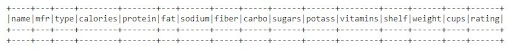There have been no null values this time. As a result, an empty data frame appears. The 9 essential functions for effective data processing have been explained. The Python and SQL languages are combined in these PySpark routines.

### PySpark Training Certification

• Lifetime LMS & Faculty Access
• Real-world & Project Based Learning

### Creation of Pyspark Dataframe:

The toDF() and createDataFrame() functions in PySpark can be used to manually configure a DataFrame from an existing RDD, range, or DataFrame. Both of these methods take various identities in order to construct a DataFrame with an existing RDD, record, or DataFrame. To generate a DataFrame out of a list, we'll require the data, so let's get started by creating the data and columns we'll necessary.

`columns = ["language","users_count"]data = [("Java", "20000"), ("Python", "100000"), ("Scala", "3000")]`

### Different ways to create Pyspark Data frame:

#### 1.Create a Data Frame out of a List Collection.

We'll examine how to make a PySpark DataFrame from such a list in this section. These examples are similar to those in the previous part with RDD, except instead of using the "rdd" object to generate DataFrame, we are using the list data object.

• Using Spark Session's createDataFrame():
Another option to manually generate PySpark DataFrame is to call createDataFrame() from SparkSession, which takes a list object as an argument. To give the names of the column, use toDF() in a chain.
`dfFromData2 = spark.createDataFrame(data).toDF(*columns)`
• Create PySpark DataFrame from an inventory of rows
In PySpark, createDataFrame() provides a second signature that takes a collection of Row types and a template for column names as parameters. To make use of this, we must first change our "data" entity from a listing to a list of Rows.
`row Data = map(lambda x: Row(*x), data) dfFromData3 = spark.createDataFrame(rowData,columns)`
• Create PySpark Data Frame with an explicit schema

You should establish the Struct Type model first then allocate it when creating a Data Frame if you want to define column names and data types.

from pyspark.sql.types import StructType,StructField, StringType, IntegerType

`data2 = [("James","","Smith","36636","M",3000),    ("Michael","Rose","","40288","M",4000),    ("Robert","","Williams","42114","M",4000),    ("Maria","Anne","Jones","39192","F",4000),    ("Jen","Mary","Brown","","F",-1)  ]schema = StructType([ \    StructField("firstname",StringType(),True), \    StructField("middlename",StringType(),True), \    StructField("lastname",StringType(),True), \    StructField("id", StringType(), True), \    StructField("gender", StringType(), True), \    StructField("salary", IntegerType(), True) \  ]) df = spark.createDataFrame(data=data2,schema=schema)df.printSchema()df.show(truncate=False)`

The output is as follows,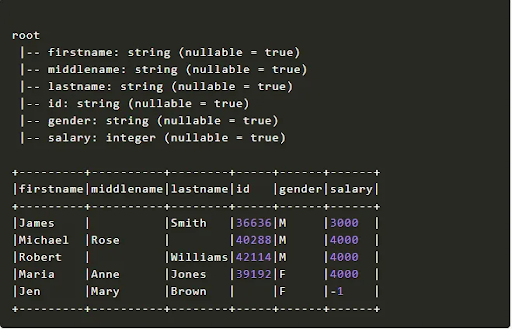#### 2. Create PySpark DataFrame from RDD

An existing RDD is a simple approach to manually generate a PySpark DataFrame. To begin, invoke the parallelize() function within SparkContext to generate a Spark RDD out of a collecting List. All of the examples that follow will require this rdd object.

`spark = SparkSession.builder.appName('SparkByExamples.com').getOrCreate()rdd = spark.sparkContext.parallelize(data)`

Using toDF() function

The toDF() method of PySpark RDD is utilized to construct a DataFrame from an existing RDD. Because RDD lacks columns, the DataFrame is generated with the predefined column names "_1" and "_2" to represent the two columns that we have.

`dfFromRDD1 = rdd.toDF()dfFromRDD1.printSchema()`

printschema() yields the below output.

`root |-- _1: string (nullable = true) |-- _2: string (nullable = true)`If you need to give column names to the DataFrame, you can use toDF() function using column names as arguments as given below.

`columns = ["language","users_count"]dfFromRDD1 = rdd.toDF(columns)dfFromRDD1.printSchema()This returns the DataFrame's schema, along with column names.Root |-- language: string (nullable = true) |-- users: string (nullable = true)`

The data type of all these columns implies the sort of data by default. This behavior can be changed by providing schema, which allows us to declare a data type, column name, and nullability for each field/column.

Using SparkSession's create DataFrame()

Another option to construct manually is to use createDataFrame() from SparkSession, which uses an rdd object as just an argument. To give the names of the columns, use toDF() in a chain.

`dfFromRDD2 = spark.createDataFrame(rdd).toDF(*columns)`

#### 3. Create Data Frame from Data sources

DataFrames are typically created in real-time from data source files such as CSV, Text, JSON, XML, and so on.

Without importing any libraries, PySpark supports a wide range of data types out of the box, and to create a DataFrame, you must use the relevant method in the DataFrameReader class.

• Create PySpark DataFrame from CSV
To generate a DataFrame from a CSV file, use the DataFrameReader object's csv() function. You could also specify options such as the delimiter for using, whether or not the data is quoted, infer schema, date formats, and so on.
`df2 = spark.read.csv("/src/resources/file.csv")`
• Create PySpark DataFrame from a Text file
Similarly, you can create a DataFrame by reading a Text file using the DataFrameReader's text() method.
`df2 = spark.read.text("/src/resources/file.txt")`
• Create PySpark DataFrame from JSON
PySpark is also capable of processing semi-structured data files such as JSON. To read a JSON file into a DataFrame, use the DataFrameReader's json() function. Here's an easy example.
`df2 = spark.read.json("/src/resources/file.json")`

#### 4. Create PySpark DataFrame from DataFrame Using Pandas

This feature was first implemented in Spark version 2.3.1. As a result, you may leverage Spark's pandas capabilities. I usually use it when I need need to do a groupBy function on a Spark dataframe or to construct rolling features and prefer to utilize Pandas rolling operations functions instead of Spark window functions.

The F.pandas udf decorator is used to implement it. We're going to assume that the function's input is a pandas data frame. In turn, we need this technique to generate a pandas dataframe.

The only complication is that we must supply a schema for the Dataframe output. To generate the outSchema, we can use the data frame's original schema.

### PySpark Training Certification

Weekday / Weekend Batches

Conclusion:

In this article, we covered the different functions of Pyspark Dataframe like select(), withColumn(), groupBy(), orderBy(), lit(), split(), etc. We also discussed how to create Pyspark Dataframe from an explicit schema, inventory rows, RDD data sources, pandas, etc.

Related Articles:

#### Find our upcoming PySpark Training Certification Online Classes

• Batch starts on 9th Feb 2023, Weekday batch

• Batch starts on 13th Feb 2023, Weekday batch

• Batch starts on 17th Feb 2023, Fast Track batch